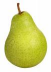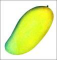Grade 7 - Data-Handling-Probability-Statistics

Choose correct answer(s) from given choice

1)Following figure shows fruits in a basket at Edward's house. Edward is getting late for school, and just picks a fruit from the basket without looking at it. Which fruit is most likely to be picked ?a. Pear b. Orange c. Mango d. Can not be determined
2)Sharon throws a die. What is the probability that she rolls a number less than 3?
a.
 5 6

b.
 3 6

c.
 1 6

d.
 2 6

3)If there are n numbers of which one isand all the others are 1's, then by how much is the arithmetic mean of these numbers less than 1.
a.
 1 n5

b. n4
c. n5 d.
 1 n4

4)John is getting dressed to go to his office. He has 6 pair of yellow socks and 8 pairs of grey socks. Without looking he reaches into the cupboard and picks a yellow sock. What is the probability that the next sock he pulls out will be of the same color?
a.
 12 27

b.
 6 14

c.
 11 27

d.
 11 28

5)If an event will occur with a probability of
 2 26
, then we can say that it is:
 a. will always occur b. less likely to occur c. cannot occur d. likely to occur
6)From a deck of cards, Laura withdraws a card at random. What is the probability that the number on the card is a prime number?

a.
 16 52

b.
 1 2

c.
 1 11

d.
 18 52

7)A teacher measures the heights of students in her class as follows (in centimetres)
��110,125,122,129,130,148,127,129,135,124,121,133,132
What is the median of their heights?
 a. 129 b. 132 c. 125 d. 133
8)An archer shot at the target 80 times and missed it 26 times. From this, can you figure out what the probability of his hitting the target with the next arrow is.
a.
 54 80

b.
 26 54

c. 1 d.
 20 80

Fill in the blanks

 9) The mean value of all the internal angles in a pentagon is �
 10) Iqbals is part of the school cricket team, and this year he has scored an average of 37 runs. He has played 6 innings so far, and his scores in 5 of them are27,29,35,46,44His score in the last innings is